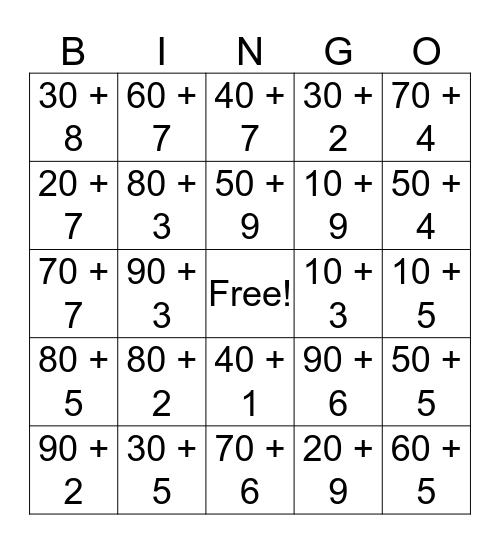# Expanded Form BingoThis bingo card has a free space and 24 words: 80 + 2, 30 + 5, 50 + 9, 90 + 2, 70 + 4, 30 + 8, 20 + 7, 60 + 7, 10 + 3, 40 + 1, 10 + 9, 70 + 7, 90 + 6, 50 + 5, 80 + 3, 20 + 9, 10 + 5, 40 + 7, 50 + 4, 90 + 3, 60 + 5, 80 + 5, 30 + 2 and 70 + 6.

## Play Online